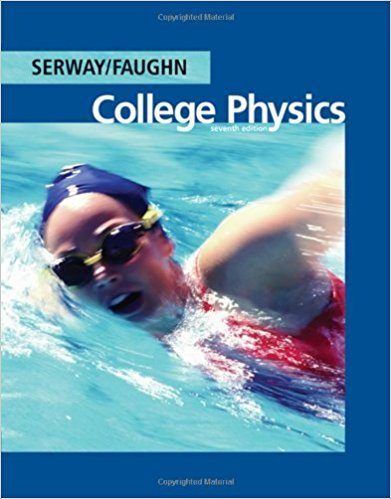×
Get Full Access to College Physics - 7 Edition - Chapter 18 - Problem 18.41
Get Full Access to College Physics - 7 Edition - Chapter 18 - Problem 18.41

×

# Assume that a length of axon membrane of about 10 cm isISBN: 9780495113690 154

## Solution for problem 18.41 Chapter 18

College Physics | 7th Edition

• Textbook Solutions
• 2901 Step-by-step solutions solved by professors and subject experts
• Get 24/7 help from StudySoup virtual teaching assistantsCollege Physics | 7th Edition

4 5 1 313 Reviews
14
4
Problem 18.41

Assume that a length of axon membrane of about 10 cm is excited by an action potential (length excited nerve speedpulse duration 50 m/s2.0 ms 10 cm). In the resting state, the outer surface of the axon wall is charged positively with K ions and the inner wall has an equal and opposite charge of negative organic ions, as shown in Figure P18.41. Model the axon as a parallel-plate capacitor, and take C 0A/d and Q CV to investigate the charge as follows: Use typical values for a cylindrical axon of cell wall thickness d 1.0108 m, axon radius r 10m, and cell-wall dielectric constant 3.0. (a) Calculate the positive charge on the outside of a 10-cm piece of axon when it is not conducting an electric pulse. How many K ions are on the outside of the axon? Is this a large charge per unit area? [Hint: Calculate the charge per unit area in terms of the number of angstroms (2) per electronic charge. An atom has a cross section of about 12 (1 1010 m)] (b) How much positive charge must ow through the cell membrane to reach the excited state of 30mV from the resting state of 70mV? How many sodium ions (Na) is this? (c) If it takes 2.0ms for the Na ions to enter the axon, what is the average current in the axon wall in this process? (d) How much energy does it take to raise the potential of the inner axon wall to 30 mV, starting from the resting potential of 70 mV?

Step-by-Step Solution:
Step 1 of 3

Step 2 of 3

Step 3 of 3

##### ISBN: 9780495113690

Unlock Textbook Solution